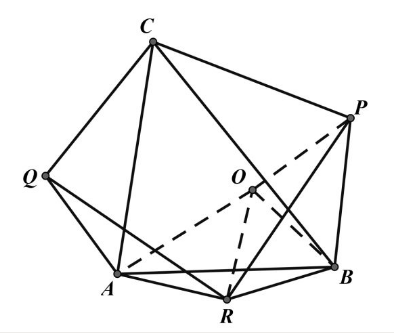【答案】 简证: 以 $B R$ 为边作正三角形 (如图)

$\triangle O A B \sim \triangle P C B, \triangle O B P \sim \triangle A B C$
$\triangle O R P \cong \triangle A R Q$
$\therefore R Q=R P, R Q \perp R P$